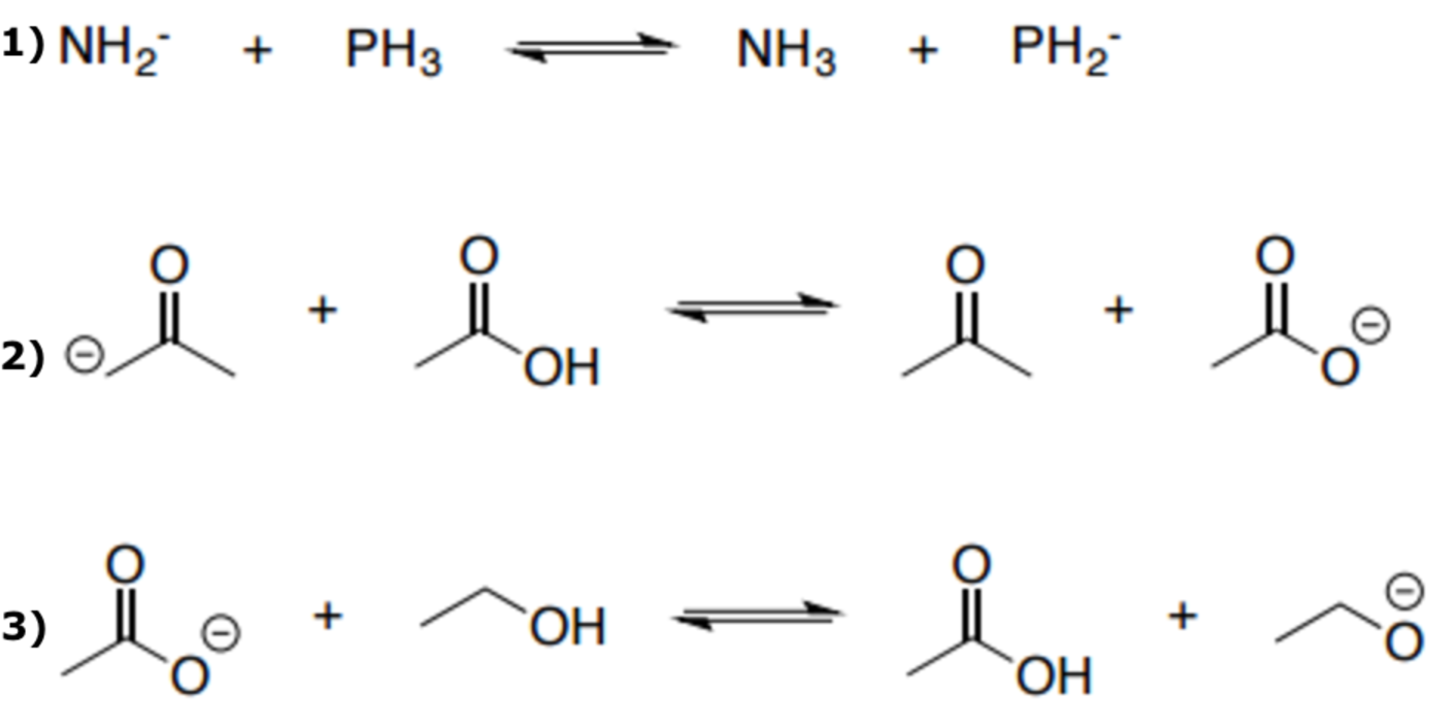# Problem: Determine the side of the reaction that is favored for each reaction: Following letters express the direction of equilibrium: R = Right L = Left

🤓 Based on our data, we think this question is relevant for Professor Anglin's class at ARIZONA.

###### Problem Details

Determine the side of the reaction that is favored for each reaction:

Following letters express the direction of equilibrium:

R = Right

L = LeftWhat scientific concept do you need to know in order to solve this problem?

Our tutors have indicated that to solve this problem you will need to apply the Acid Base Equilibrium concept. You can view video lessons to learn Acid Base Equilibrium. Or if you need more Acid Base Equilibrium practice, you can also practice Acid Base Equilibrium practice problems.

What is the difficulty of this problem?

Our tutors rated the difficulty ofDetermine the side of the reaction that is favored for each ...as medium difficulty.

How long does this problem take to solve?

Our expert Organic tutor, Jonathan took 2 minutes and 33 seconds to solve this problem. You can follow their steps in the video explanation above.

What professor is this problem relevant for?

Based on our data, we think this problem is relevant for Professor Anglin's class at ARIZONA.